Multiplication/Word Problems
Common denominators
Equivalent fractions
Compare fractions
100

10 X 8=

80

100

What is the common denominator of (1/2) and (1/4)?

4, 8, 12, etc

100

A part of a whole

a half

100

Two or more fractions that name the same amount

Equivalent fractions
100

Make the statement true.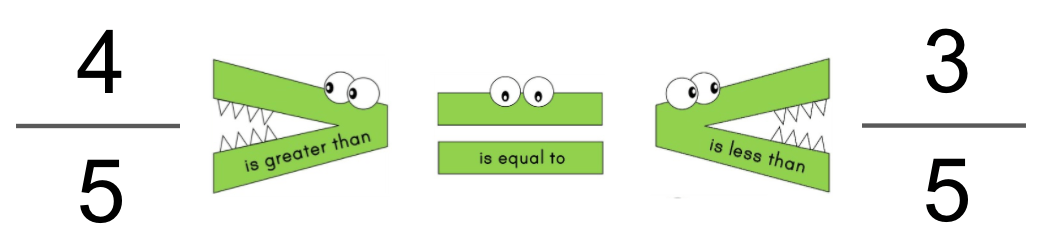greater than

200

There are 4 students in the classroom. Each student has 1 pencil. How many pencils are there in all?  Give the multiplication sentence with the answer

4 x 1 = 4

200

What is the common denominator of (1/4) and (5/6)?

12, 24

200

The top number of a fraction is called the ______ and the bottom number of a fraction is called the ______.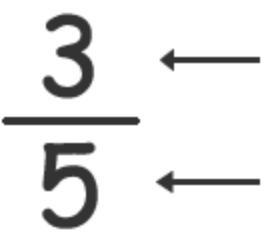Numerator for the top number and denominator for the top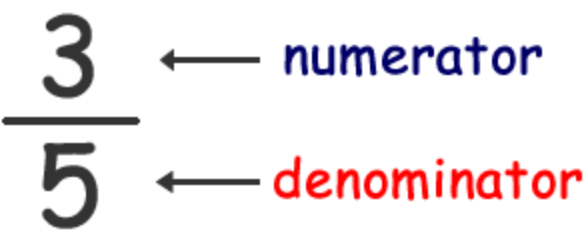200

What is the equivalent fraction?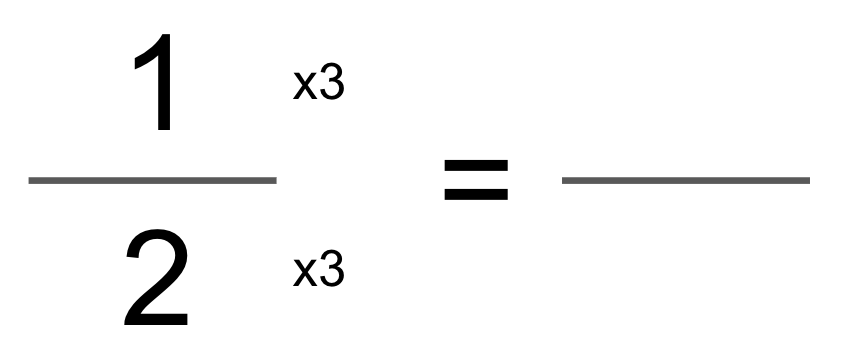3/6 or 1/2

200

Make the statement true.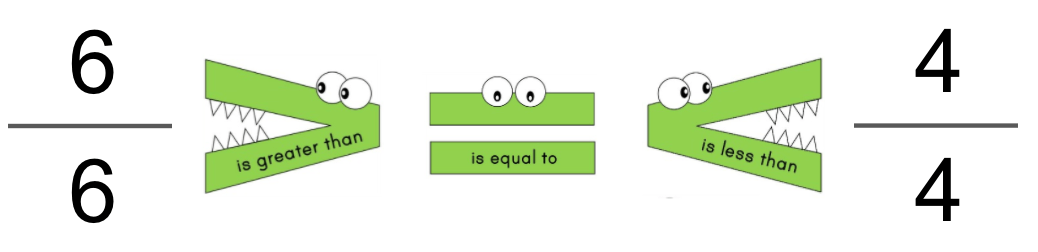equal

=

300

5 athletes ran 3 laps each. How many laps were run in all by the athletes? Give the multiplication sentence with the answer

5 x 3 = 15

300

What is the common denominator of (2/3) and (7/9)?

18, 27

300

Any fraction that has the same number in the numerator and denominator is equal to...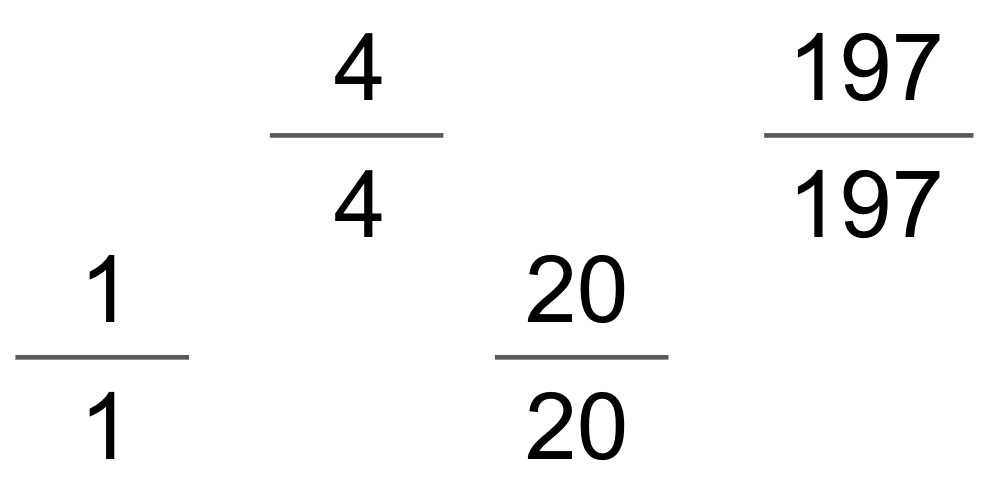1 or one whole

300

How is the equivalent fraction made?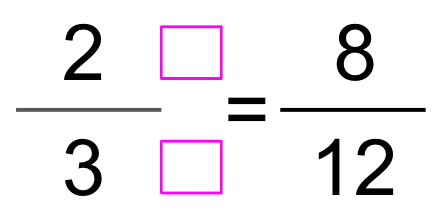4

300

Make the statement true.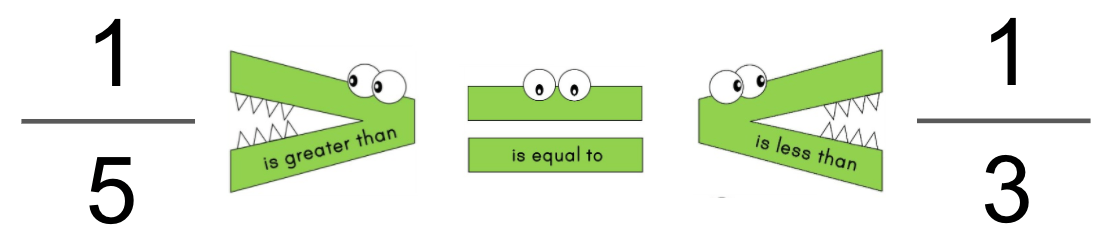less than

<

400

36x50

1800

400

What is the common denominator of (9/15) and (4/9)?

45

400

In a fraction, the number of equal parts the whole is divided into is the...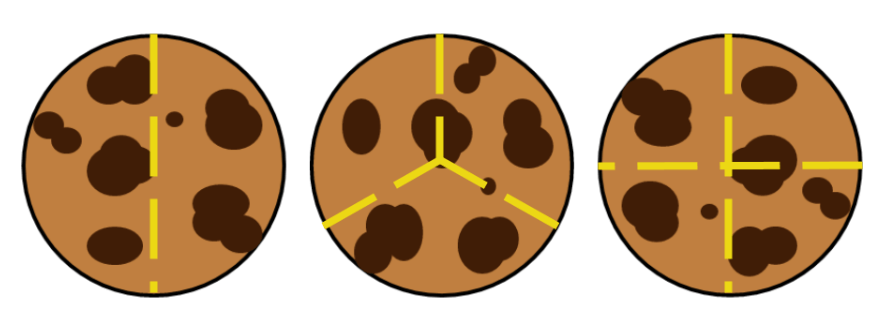denominator

400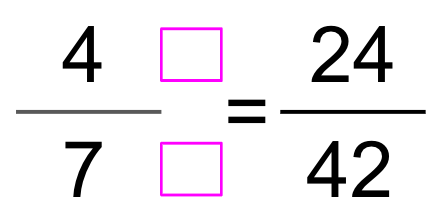x 6

400

Make the statement true.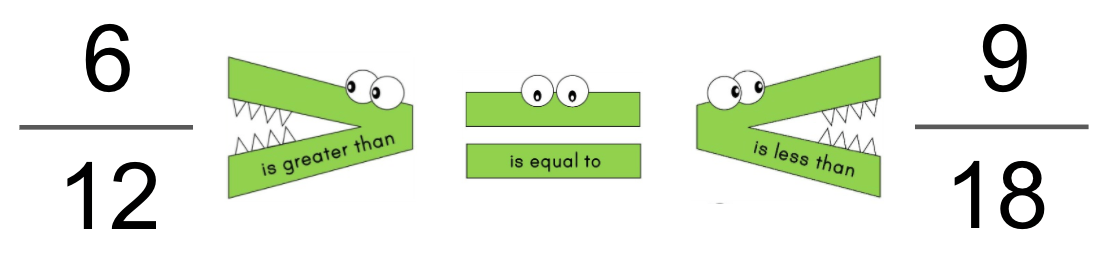=

500

There were 30 presents in all. 5 adults brought the same number of presents. How many presents did each adult bring?  Give the multiplication sentence with the answer

5 x 6 =30

500

What is the common denominator of 1/6 and 3/4?

12, 24

500

As the denominator increases, the size of the piece...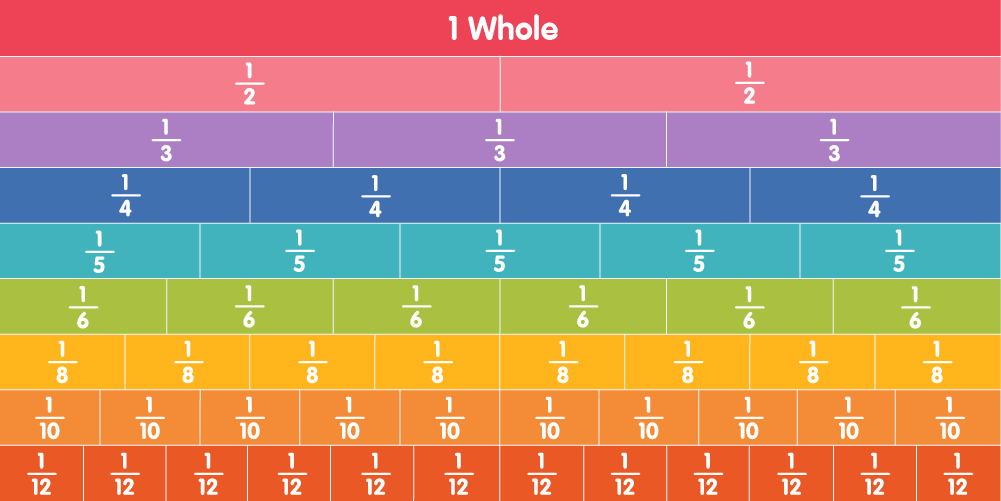decreases

500

Make a fraction that is equivalent to the fraction below.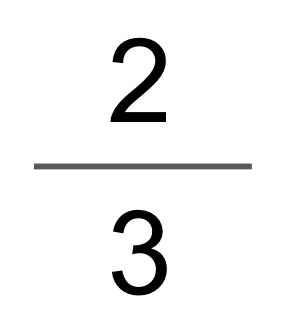4/6, 6/9, etc

500

Make the statement true.less than <

Click to zoom